Rg-Calculator of Polyhedra

Selected Polyhedra can consist of up to 3 different types of faces (equilateral n-polygonal or rhombic). Enter the type and number of the (different) polygonal faces, their common (equilateral) edge length (a) and either their common out-sphere(ro), mid-sphere(rm) or in-sphere(ri) radius originating in the centroid of the respective polyhedron, extending to the centroid of the faces (ri), of the edges (rm) or to the vertices (ro), respectively. In case of rhombic faces only the in-sphere-radius (ri) can be entered together with the ratio of the rhombic axes (0 < rx/ry < 1). The volume, surface, perimeter (sum of edge lengths), Radius of Gyration of the solid (Rgs), of the faces (Rgf), of the edges (Rge) and of the vertices (Rgv) will be calculated for the entered parameters and also for the same shape where the volume is normalized to 1. Units are arbitrary.

a: rx/ry:
n:
n:
n:

 Volume: Surface: Perimeter: Rg (solid): Rg (faces) Rg (edges): Rg (vertices):
 Volume: Surface: Perimeter: Rg (solid): Rg (faces) Rg (edges): Rg (vertices):

Radius of Gyration (Rg) of the 5 Platonic Solids

The values for the Volume (V), the Surface (S), the out-sphere (circum-sphere) radius (ro), the mid-sphere radius (rm), the in-sphere radius (ri), the Radius of Gyration of the solid (Rgs), of the faces (Rgf) and of the edges (Rge), the number and type of polygonal faces (n x p), number of edges (e) and vertices (v) of the 5 Platonic Solids with edge length a = 1 are compiled in the following table. Units are arbitrary.

 Solid Volume Surface ro rm ri Rg(s) Rg(f) Rg(e) faces e v Tetrahedron 0.11785 1.73205 0.61237 0.35355 0.20412 0.27386 0.35355 0.45644 4x3p 6 4 Hexahedron 1.00000 6.00000 0.86603 0.70711 0.50000 0.50000 0.64550 0.76376 6x4p 12 8 Octahedron 0.47140 3.46410 0.70711 0.50000 0.40825 0.38730 0.50000 0.57735 8x3p 12 6 Dodecahedron 7.66312 20.64573 1.40126 1.30902 1.11352 0.95448 1.23223 1.34047 12x5p 30 20 Icosahedron 2.18170 8.66025 0.95106 0.80902 0.75576 0.62666 0.80902 0.85898 20x3p 30 12

Radius of Gyration (Rg) of the 13 Archimedean Solids and of their Duals (Catalan Solids).

The values for the Radius of Gyration of the solid (Rgs), of the faces (Rgf), of the edges (Rge) and of the vertices (Rgv) of the 13 Archimedean Solids and their corresponding Duals, the Catalan Solids, all with their respective volume normalized to V=1, are compiled in the following table, together with the number of the faces (f), edges (e) and vertices (v) for each polyhedron. For comparison, also the Rg-values for a sphere with the same volume (V=1) are given. Units are arbitrary. Source of shape images: Wikipedia.

 Shape Archimedean Solid Rg(s) Rg(f) Rg(e) Rg(v) f e v Rg(v) Rg(e) Rg(f) Rg(s) Dual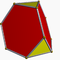Truncated Tetrahedron 0.51643 0.65705 0.78839 0.84100 8 18 12 0.84671 0.75184 0.65299 0.50581 Triakis Tetrahedron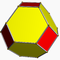Truncated Octahedron 0.48542 0.62564 0.68044 0.70432 14 36 24 0.71487 0.66002 0.62412 0.48344 Tetrakis Hexahedron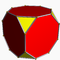Truncated Cube 0.49486 0.63546 0.72534 0.74523 14 36 24 0.71843 0.68337 0.63082 0.48863 Triakis Octahedron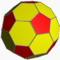Truncated Icosahedron 0.48105 0.62099 0.64158 0.65047 32 90 60 0.65772 0.63364 0.62077 0.48085 Pentakis Dodecahedron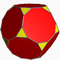Truncated Dodecahedron 0.48362 0.62373 0.66884 0.67526 32 90 60 0.65267 0.64373 0.62229 0.48203 Triakis Icosahedron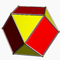Cuboctahedron 0.48697 0.62740 0.68594 0.75141 14 24 12 0.73482 0.68736 0.62748 0.48604 Rhombic Dodecahedron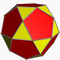Icosidodecahedron 0.48231 0.62212 0.65219 0.67399 32 60 30 0.66228 0.64641 0.62176 0.48161 Rhombic Triacontahedron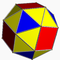Snub Cube 0.48151 0.62142 0.64307 0.67498 38 60 24 0.66308 0.64913 0.62161 0.48150 Pentagonal Icositetrahedron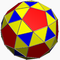Snub Dodecahedron 0.48088 0.62066 0.63177 0.64341 92 150 60 0.63613 0.63208 0.62062 0.48073 Pentagonal Hexecontahedron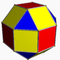Small Rhombicuboctah. 0.48173 0.62180 0.65024 0.67983 26 48 24 0.67536 0.65001 0.62176 0.48161 Deltoidal Icositetrahedron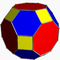Great Rhombicuboctah. 0.48308 0.62273 0.65737 0.66781 26 72 48 0.66544 0.64201 0.62193 0.48174 Disdyakis Dodecahedron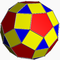Small Rhombicosidodecah. 0.48081 0.62066 0.63350 0.64436 62 120 60 0.64153 0.63206 0.62061 0.48072 Deltoidal Hexecontahedron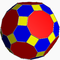Great Rhombicosidodecah. 0.48137 0.62107 0.63927 0.64299 62 180 120 0.63669 0.62992 0.62077 0.48085 Disdyakis Triacontahedron Shape Dual Rg(s) Rg(f) Rg(e) Rg(v) v e f Rg(v) Rg(e) Rg(f) Rg(s) Catalan Solid - Sphere 0.48052 0.62035 0.62035 0.62035 0 0 0 0.62035 0.62035 0.62035 0.48052 Sphere

Author: M.Kriechbaum, TU-Graz (2014), e-mail: manfred.kriechbaum@tugraz.at